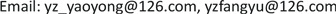OJCSOpen Journal of Circuits and Systems2327-0853Scientific Research Publishing10.12677/OJCS.2019.82002OJCS-29794OJCS20190200000_82554710.pdf信息通讯 工程技术 一种单相光伏并网逆变器软件锁相环的设计与实现 Design and Implementation of a Software Phase-Locked Loop for Single-Phase PV Grid-Connected Inverter 2121亚华21明南21继勇31扬州大学，信息工程学院，江苏 扬州扬州大学，水利与能源动力工程学院，江苏 扬州null190420190802515© Copyright 2014 by authors and Scientific Research Publishing Inc. 2014This work is licensed under the Creative Commons Attribution International License (CC BY). http://creativecommons.org/licenses/by/4.0/

1扬州大学，信息工程学院，江苏 扬州

2扬州大学，水利与能源动力工程学院，江苏 扬州Copyright © 2019 by author(s) and Hans Publishers Inc.

This work is licensed under the Creative Commons Attribution International License (CC BY).

http://creativecommons.org/licenses/by/4.0/1. 引言

2. 软件PLL原理2.1. PLL原理

{ v d = v α ⋅ cos θ + v β ⋅ sin θ v q = v β ⋅ cos θ − v α ⋅ sin θ (1)

θ表示锁相环得到的相位角，vgrid表示电网电压。

{ v d = v grid ⋅ cos θ v q = − v grid ⋅ sin θ (2)

{ v d = V grid ⋅ sin φ ⋅ cos θ v q = − V grid ⋅ sin φ ⋅ sin θ (3)

{ v d = V grid 2 ⋅ [ sin ( φ − θ ) + sin ( φ + θ ) ] v q = V grid 2 ⋅ [ cos ( φ + θ ) − cos ( φ − θ ) ] (4)

(5)

d v q d t = d v q d φ ⋅ d φ d t + d v q d θ ⋅ d θ d t (7)

d v q d t = V grid 2 ⋅ [ − sin ( φ + θ ) ] ⋅ ( 2 ⋅ ω f f ) (8)

ω e r r = d v q d t ⋅ 1 2 ω f f + v d = V grid 2 ⋅ sin ( φ − θ ) (9)

2.2. 软件PLL的实现方法

vq对时间的微分在程序中是这样实现的：在s域，vq对时间的微分可以用 s ⋅ v q 表示，对于 s ⋅ v q 进行离散化可以得到如下式子：

(10)

1 2 ω f f G ( z ) = f s 2 ω f f ⋅ ( v q − v q ⋅ z − 1 ) (11)

f s 2 ω f f ( v q ( k ) − v q ( k − 1 ) ) (12)

3. PLL仿真3.1. 软件PLL仿真

3.2. 双通道微逆变器的仿真实现软件PLL算法

3.3. H6桥逆变器中的软件PLL算法

4. 实验结果与分析